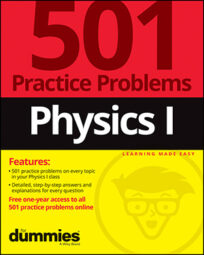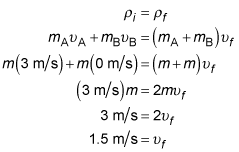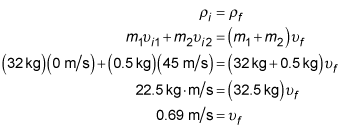##### Physics I: 501 Practice Problems For Dummies (+ Free Online Practice)Using physics, you can calculate how fast two objects will move after an inelastic collision. For example, you can calculate how fast a skater moves after catching a snowball.

Here are some practice questions that you can try.

## Practice questions

1. Object A rams into object B at a speed of 3 meters per second in a perfectly inelastic collision. Object B was originally motionless, and both A and B have the same mass. How fast are the objects moving after the collision?

Round your answer to the nearest tenth of a meter per second.

2. A stationary, 32-kilogram ice skater catches a 0.5-kilogram snowball thrown at a speed of 45 meters per second. How fast — in meters per second — does the skater move afterward?

The following are the answers to the practice questions:

1. 1.5 m/s

The collision is perfectly inelastic, so objects A and B will stick together after the collision and have the same velocity. Mass and velocity are inversely related in the formula for momentum, which is conserved in collisions. The mass of the moving object is increased by a factor of 2 from before the collision to after the collision, so the velocity must decrease by a factor of 2.

If you're more comfortable working with the equation, let each object's mass be labeled something generic — like m — and solve the conservation-of-momentum equation for the final velocity:2. 0.69 m/s

This is an example of a perfectly inelastic collision because the skater catches the snowball and doesn't let it bounce off; after the collision, only one object is moving. If you label the original direction of the snowball as positive and the skater as object 1, and then substitute values, you get